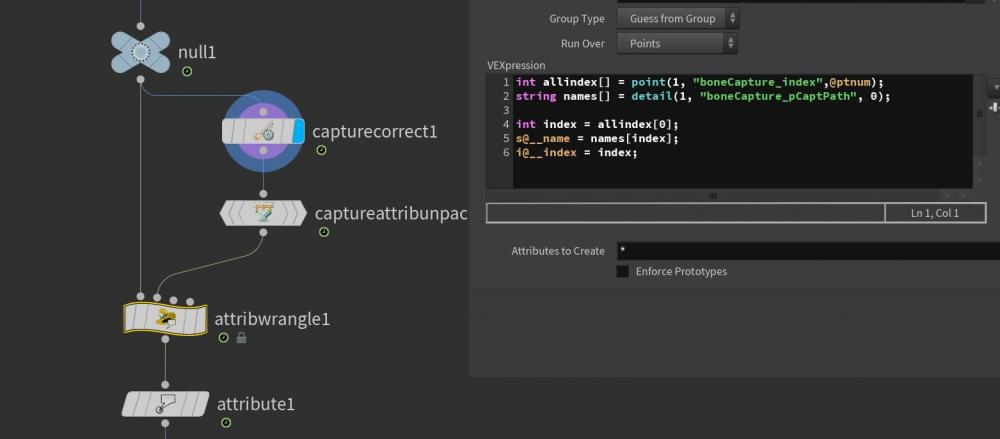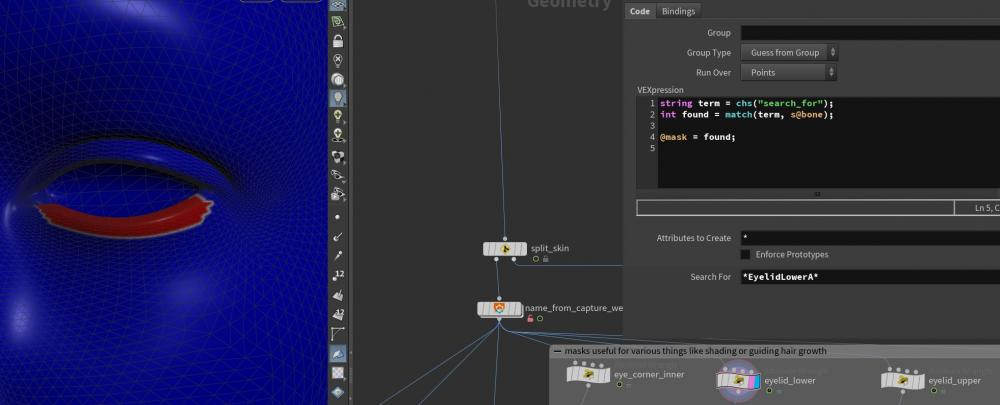# extract capture weights per bone to point attribute

## Recommended Posts

Hi all,

I'm working with a character .fbx imported from another package, and need to extract the painted weights for a specific bone to a point attribute to use as a mask. Right now i'm using the labs Name from Capture Weight node, which unpacks the weights and saves the name of the bone that has the highest influence on a point to string attribute by just returning the first entry. Then I use the following vex to do a search for a particular bone(s) in that string attribute, something like "eyelidLower*":

```string term = chs("search_for");
int found = match(term, s@bone);

that returns 1 for all the points that match the term so i can a linear mask, but I want to return and sum the actual weights - is this possible?

Many thanks##### Share on other sites

```//reduce
int maxInf = (int)chf("maxInfluence");

f[]@boneCapture_data;
i[]@boneCapture_index;

int length = len( @boneCapture_data ) - maxInf;

if(length > 0){
for(int i = 0; i < length; i++)
{
removeindex( @boneCapture_data, argsort(@boneCapture_data) );
removeindex( @boneCapture_index, argsort(@boneCapture_data) );
}
}```
```//norml
f[]@boneCapture_data;
float scaleFactor = 1.0 / sum(@boneCapture_data);

for(int i = 0; i < len(@boneCapture_data); i++)
{
@boneCapture_data[i] = @boneCapture_data[i] * scaleFactor;
}```
```//round
float accuracy = 1.0 / chf("accuracy");

f[]@boneCapture_data;

for(int i = 0; i < len(@boneCapture_data); i++)
{
@boneCapture_data[i] = rint (@boneCapture_data[i] * accuracy ) / accuracy;
}

int maxIdx = reverse( argsort(@boneCapture_data) );
@boneCapture_data[maxIdx] = @boneCapture_data[maxIdx] + ( 1.0 - sum(@boneCapture_data) );
```
```//addsource
string sourceBone = ch("sourceBone");
string targetBone = ch("targetBone");

// check bone name exist
int sourceIndex = -1;
int targetIndex = -1;
string nameList[] = detail(0, "boneCapture_pCaptPath");
for(int i = 0; i < len(nameList); i++)
{
if(nameList[i] == sourceBone){
sourceIndex = i;
}
if(nameList[i] == targetBone){
targetIndex = i;
}
if(sourceIndex != -1 && targetIndex != -1){
break;
}
}

if( sourceIndex == -1 || targetIndex == -1){
// do nothing when bone name is invailed
return;
}
else{
f[]@boneCapture_data;
i[]@boneCapture_index;
int source = -1;
int target = -1;
for(int i = 0; i < len(@boneCapture_index); i++)
{
if(@boneCapture_index[i] == sourceIndex){
source = i;
}
if(@boneCapture_index[i] == targetIndex){
target = i;
}
if(source != -1 && target != -1){
break;
}
}

// update weight value;
if(source != -1 && target == -1){
@boneCapture_index[source] = targetIndex;
}
if(source != -1 && target != -1){
@boneCapture_data[target] = @boneCapture_data[target] + @boneCapture_data[source];
removeindex( @boneCapture_data, source );
removeindex( @boneCapture_index, source );
}
}```
```//dell anused
string boneNames[] = detail(0, "boneCapture_pCaptPath");
float boneXforms[] = detail(0, "boneCapture_pCaptData");
string captNames[] = detail(0, "capt_names");
float captXforms[] = detail(0, "capt_xforms");
int captParents[] = detail(0, "capt_parents");

string boneNamesKeep[] = {"root", "thumb_03_r"};

// check unused bone name list
int boneNames_Used[];
for(int i = 0; i < @numpt; i++){
int ptBone[] = point(0, "boneCapture_index", i);
for(int j = 0; j < len(ptBone); j++){
boneNames_Used[ ptBone[j] ] = 1;
}
}
for(int i = 0; i < len(boneNamesKeep); i++){
for(int j = 0; j < len(boneNames); j++){
if(boneNamesKeep[i] == boneNames[j]){
boneNames_Used[ j ] = 1;
}
}
}

// remove unused bone name list
int originalIdx[];
int oIdxCount = 0;
for(int i = (len(boneNames) - 1) ; i >= 0; i--){
if(boneNames_Used[i] == 0){
removeindex(boneNames, i);
}
else{
originalIdx[oIdxCount] = i;
oIdxCount++;
}
}
originalIdx = reverse(originalIdx);

// update bone Xform, parent list
float newBoneXforms[];
float newCaptXforms[];
int newCaptParents[];
for(int i = 0; i < len(originalIdx); i++){
int bIdx = originalIdx[i] * 20;
int cIdx = originalIdx[i] * 16;
append(newBoneXforms, boneXforms[bIdx:bIdx+20]);
append(newCaptXforms, captXforms[cIdx:cIdx+16]);

newCaptParents[i] = captParents[ originalIdx[i] ];
}

// update parent index
for(int i = 0; i < len(newCaptParents); i++){
int check = 0;
for(int j = 0; j < len(originalIdx); j++){
if(newCaptParents[i] == originalIdx[j]){
newCaptParents[i] = j;
check = 1;
break;
}
}
if(check == 0){
newCaptParents[i] = -1;
}
}

// update bone index of point
for(int i = 0; i < @numpt; i++){
int ptBone[] = point(0, "boneCapture_index", i);
for(int j = 0; j < len(ptBone); j++){
for(int k = 0; k < len(originalIdx); k++){
if(ptBone[j] == originalIdx[k]){
ptBone[j] =k;
break;
}
}
}
setpointattrib(0, "boneCapture_index", i, ptBone);
}

// update attriute
s[]@boneCapture_pCaptPath = boneNames;
f[]@boneCapture_pCaptData = newBoneXforms;
s[]@capt_names = boneNames;
f[]@capt_xforms = newCaptXforms;
i[]@capt_parents = newCaptParents;```
```//swap source
string sourceBone = ch("sourceBone");
string targetBone = ch("targetBone");

// check bone name exist
int sourceIndex = -1;
int targetIndex = -1;
string nameList[] = detail(0, "boneCapture_pCaptPath");
for(int i = 0; i < len(nameList); i++)
{
if(nameList[i] == sourceBone){
sourceIndex = i;
}
if(nameList[i] == targetBone){
targetIndex = i;
}
if(sourceIndex != -1 && targetIndex != -1){
break;
}
}

if( sourceIndex == -1 || targetIndex == -1){
// do nothing when bone name is invailed
return;
}
else{
f[]@boneCapture_data;
i[]@boneCapture_index;
int source = -1;
int target = -1;
for(int i = 0; i < len(@boneCapture_index); i++)
{
if(@boneCapture_index[i] == sourceIndex){
source = i;
}
if(@boneCapture_index[i] == targetIndex){
target = i;
}
if(source != -1 && target != -1){
break;
}
}

// update weight value;
if(source != -1 && target == -1){
@boneCapture_index[source] = targetIndex;
}
if(source == -1 && target != -1){
@boneCapture_index[target] = sourceIndex;
}
if(source != -1 && target != -1){
float targetWeight = @boneCapture_data[target];
@boneCapture_data[target] = @boneCapture_data[source];
@boneCapture_data[source] = targetWeight;
}
}```
##### Share on other sites

• 1 month later...

## Join the conversation

You can post now and register later. If you have an account, sign in now to post with your account.
Note: Your post will require moderator approval before it will be visible.×   Pasted as rich text.   Paste as plain text instead

Only 75 emoji are allowed.

×   Your previous content has been restored.   Clear editor

×   You cannot paste images directly. Upload or insert images from URL.

×
×